# Time

GCSELevel 1-3AQACambridge iGCSEEdexcelEdexcel iGCSEOCRWJEC

## Time

Time is a very important measurement.

Time can be recorded in many different ways:

• $12$ hour clocks
• $24$ hour clocks
• Analogue clocks
• Digital clocks
• Timetables
Level 1-3GCSEAQAEdexcelOCRWJECCambridge iGCSEEdexcel iGCSE

## Units of Time

Time has various different units, the majority of which you should already be familiar with.

In your exam, you may need to convert between different units of time.

Shown below are some common conversions you need to know:

 $60$ seconds $=1$ minute $60$ minutes $=1$ hour $24$ hours $=1$ day $7$ days $=1$ week $365$ days $=1$ year $52$ weeks $=1$ year $12$ months $=1$ year $10$ years $=1$ decade $100$ years $=1$ century

We can also divide $1$ hour into fractions of an hour, a few examples are:

 $15$ minutes $=\frac{1}{4}$ hour $30$ minutes $=\frac{1}{2}$ hour $45$ minutes $=\frac{3}{4}$ hour
Level 1-3GCSEAQAEdexcelOCRWJECCambridge iGCSEEdexcel iGCSE

## Time Formats

Times of the day can be displayed by either a $\textcolor{blue}{12}$ hour clock, or a $\textcolor{red}{24}$ hour clock.

$\textcolor{blue}{12}$ hour clocks show times between $12$ am (midnight) and $12$ pm (midday).

$\textcolor{red}{24}$ hour clocks show times between $00:00$ (midnight) and $23:59$ (one minute to midnight the next day), with $12:00$ being midday.

For example, here are some comparisons between a $\textcolor{blue}{12}$ and $\textcolor{red}{24}$ hour clock: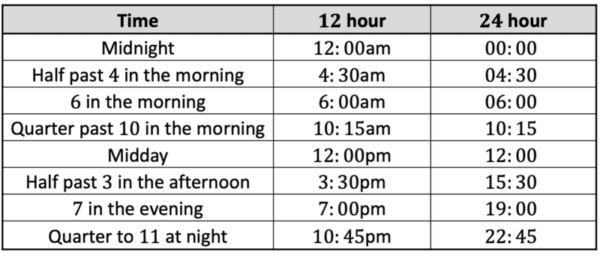Hint: if you are given a time in the afternoon in $\textcolor{red}{24}$ hour format, subtract $12$ hours to tell the time.

For example, $16:00$ subtract $12$ hours is $4:00$, so we know this is $4$ in the afternoon.

Level 1-3GCSEAQAEdexcelOCRWJECCambridge iGCSEEdexcel iGCSE
Level 1-3GCSEAQAEdexcelOCRWJECCambridge iGCSEEdexcel iGCSE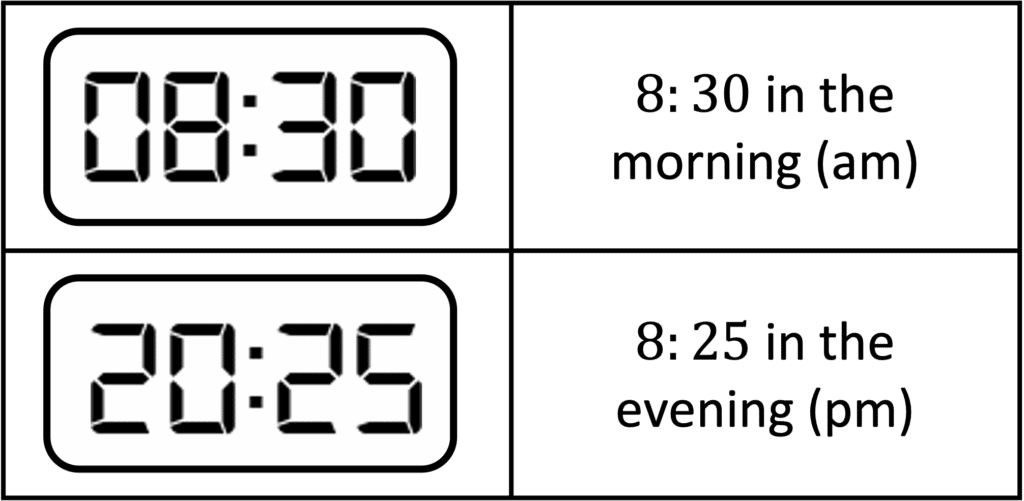## Digital Clocks

Digital clocks usual show time in $\textcolor{red}{24}$ hour format, and they are easy to understand.

The digits before the colon represent the hour, and the digits after the colon represent the minutes.

Level 1-3GCSEAQAEdexcelOCRWJECCambridge iGCSEEdexcel iGCSE

## Analogue Clocks

Analogue clocks usually show time in $\textcolor{blue}{12}$ hour format, and they are more difficult to understand.

The shorter hand represents the hour, and the longer hand represents the minutes.

Hours:

The short hand is points to the hour. If it is pointing at $10$, we know it is $10$ o’clock.

From an analogue clock, it is impossible to tell whether this time is in the morning or afternoon.

Minutes:

For minutes, we use the longer hand.

We know there are $60$ minutes in an hour, and as analogue clocks are split into $12$ sections (between each number), each section represents $5$ minutes.

For example, the gap between the $12$ and $1$ on the clock represents $5$ minutes.

We start the clock at $12$, and when the longer hand is pointing at $12$ we know this is $0$ minutes. If the longer hand was pointing at $5$, we can multiply this by $5$ minutes to work out the minutes:

$5\times5=25$

So, pointing at the $5$ would represent $25$ minutes past.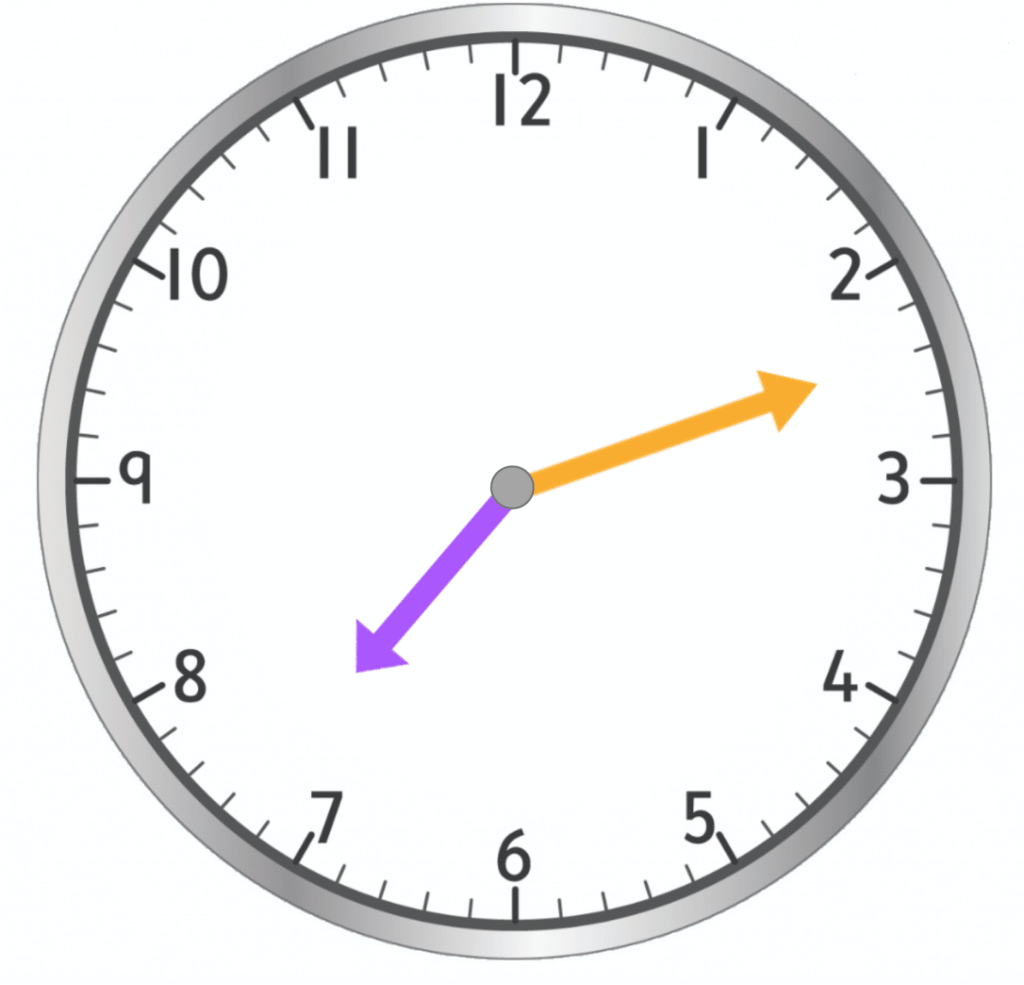Worked example: reading an analogue clock

Hours: the shorter hand is pointing between the $7$ and $8$, so we know it is somewhere between $7:00$ and $8:00$, so the hour of the day would be $7$.

Minutes: the longer hand is pointing between the $2$ and $3$. The $2$ represents $2\times5=10$ minutes, and the $3$ represents $15$ minutes. The hand is slightly closes to $2$ than $3$, so the minutes will be around $12$.

Therefore, the time is $7:12$.

Level 1-3GCSEAQAEdexcelOCRWJECCambridge iGCSEEdexcel iGCSE
Level 1-3GCSEAQAEdexcelOCRWJECCambridge iGCSEEdexcel iGCSE

## Days of the Month

Another important thing to remember is how many days each month has.

$30$ days$\begin{cases} \text{April}\\ \text{June}\\ \text{September}\\ \text{November}\\ \end{cases} \\$

$31$ days$\begin{cases} \text{January}\\ \text{March}\\ \text{May}\\ \text{July}\\ \text{August}\\ \text{October}\\ \text{December} \\ \end{cases}$

$\text{February}$ is unique, it has $28$ days normally and $29$ days in a leap year.

A leap year normally occurs once every $4$ years.

Level 1-3GCSEAQAEdexcelOCRWJECCambridge iGCSEEdexcel iGCSE

## Timetables

It is also important to be able to understand and use time from timetables.

For example, here is a train timetable: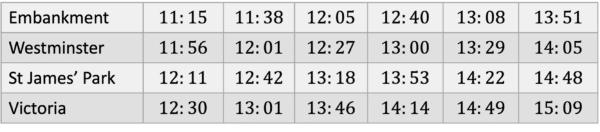The columns represent different train services: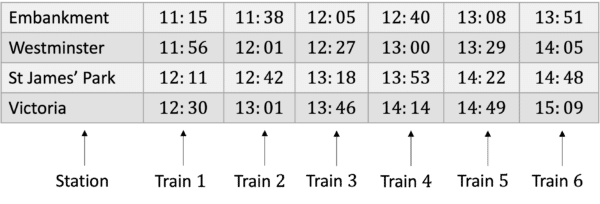Solving problems with timetables:

How long would it take to get to Victoria if the $12:05$ train was boarded from Embankment?

The train leaves at $12:05$, and looking down the column, we can see it arrives at Victoria at $13:46$.

The easiest way to calculate this is by splitting it into the $2$ hours:

$12:05$ to $13:00$ is $55$ minutes.

$13:00$ to $13:46$ is $46$ minutes.

Total $= 55 + 46 = 101$ minutes.

We can leave this in minutes, or the question may ask us for this in hours. As there are $60$ minutes in an hour:

$101-60=41$ minutes left.

The journey takes $1$ hour and $41$ minutes.

Level 1-3GCSEAQAEdexcelOCRWJECCambridge iGCSEEdexcel iGCSE
Level 1-3GCSEAQAEdexcelOCRWJECCambridge iGCSEEdexcel iGCSE

## Example 1: Analogue Clocks

Helena is starting a new job. Each day, her shift will start at the time shown on Clock $1$, and finish at the time shown on Clock $2$. How long is her shift each day?

[4 marks]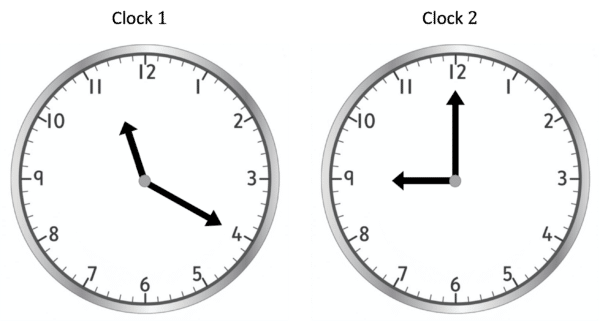Clock $1$:

Hours: $11$

Minutes: pointing at $4$, $4\times5=20$

Time: $11:20$

Clock $2$:

Hours: $9$

Minutes: pointing at $12$, which we know is $0$ minutes

Time: $9:00$

So, Helena starts work at $11:20$ and finishes at $9:00$:

Time before $12:00 - 40$ minutes

Time after $12:00 - 9$ hours.

Her shift is $9$ hours and $40$ minutes.

Level 1-3GCSEAQAEdexcelOCRWJECCambridge iGCSEEdexcel iGCSE

## Example 2: Timetables

Ellias is trying to get the bus to school. He lives by Blackfriars and his school is by Cannon Street. His school starts at $10:00$ and he wants to arrive at least $10$ minutes before school starts. What is the latest bus he could catch from Blackfriars

[2 marks]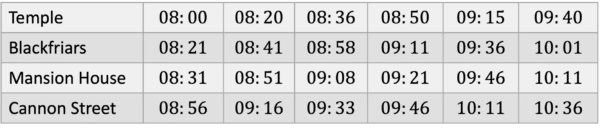To arrive $10$ minutes before school, he needs to arrive at or before $09:50$ at Cannon Street.

The bus in the fourth column arrives the closest to this time, at $09:46$.

Therefore, he should get the bus from Blackfriars at $09:11$.

Level 1-3GCSEAQAEdexcelOCRWJECCambridge iGCSEEdexcel iGCSE

## Time Example Questions

Adding $5$ hours on to $1:25$, we get $6:25$pm.

Now, to convert this to $24$ hour time, we add $12$ hours to the time.

$6:25 + 12\text{ hours} = 18:25$Gold Standard Education

He leaves at $13:48$ and it takes $25$ minutes.

Splitting this into chunks, the section of his journey before  $14:00$, will be $60-48=12$ minutes.

Remaining: $25-12=13$ minutes.

He will arrive $13$ minutes after $14:00$, at $14:13$.

His work starts at quarter past $2$ pm, which is the same as $14:15$.

Therefore, he will be on time.Gold Standard Education

Alana can either get the train at $13:58, 15:30,$ or $16:18$.

$13:58$ train arrives at $15:03$, so the time taken is:

Before $14:00$, $2$ minutes.

Between $14:00 - 15:00$, $1$ hour.

After $15:00$, $3$ minutes.

Total: $1$ hour and $2+3=5$ minutes.

$1$ hour and $5$ minutes.

$15:30$ train arrives at $16:22$, so the time taken is:

Before $16:00$, $30$ minutes.

After $16:00$, $22$ minutes.

Total: $30+22=52$ minutes.

$16:18$ train arrives at $17:13$, so the time taken is:

Before $17:00$, $42$ minutes.

After $17:00$, $13$ minutes.

Total: $42+13=55$ minutes.

Therefore, Alana should get the $15:30$ train.Gold Standard EducationProduct